# Objective questions on Electric Potential

## Paragraph Type of Question

(A) Electric potential at any point x, y, z in the space is given $V = 4x^2 - 3x$
Question 1
Find the electric field at any point (x, y, z)
(a) (8x - 3)i
(b) -(8x - 3)i
(c) -8xi
(d) 8xi

V = 4x2 - 3x
Ex = ∂V/∂x = 8x - 3
Ey = ∂V/∂y = 0
Ez = ∂V/∂z = 0
So, E = -(8x - 3)i
Hence (b) is correct

Question 2
Equipotential surface in the region are
(a) planes parallel to X-Y plane
(b) planes parallel to Y-Z plane
(c) planes parallel to X-Z plane
(d) none of the above

(b) is the correct choice

Question 3
Find the force experienced by a charge particle q at point (1, 1, 1)
(a) -11qi
(b) 11qi
(c) 11qi + j
(d) 11qi – j

E = -(8x - 3)i
F = qE = q (-8x - 3)i = q (-8 -3)i = -11qi
Hence (a) is correct

Question 4
Find the value of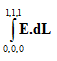a. -1 volt
b. 0 volt
c. -2 volt
d. 1 volt

$V_{(1,1,1)} – V_{(0,0,0)}= - \int_{0,0,0}^{1,1,1} \boldsymbol{E}.d\mathbf{L}$
$\int_{0,0,0}^{1,1,1} \boldsymbol{E}.d\mathbf{L}=V_{(0,0,0)}-V_{(1,1,1)} = -1 Volt$
Hence (a) is correct

(B) A spherical shell of radius R is carries a uniform surface charge q. Take the reference point at ∞
Question 5
Find the electric field at r>R .Here is the r is the unit vector across radial direction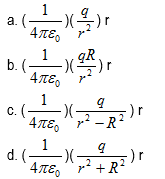From Gauss law
$\int \mathbf{E}.d\mathbf{S}$ = qin0
E × 4πr2 = q/ε0
$E =\frac {1}{4 \pi \epsilon _{0} } \frac {q}{r^2}$ radially outward
Hence (a) is correct

Question 6
Find the electric field at r<R
a. (1/4πε0) (q/R2)
b. (1/4πε0) (q/r2)
c. zero
d. (1/4πε0) (q/r2 + R2)

(c) is correct choice

Question 7
Find the potential at r>R
a. (1/4πε0) (q/r)
b. (1/4πε0) (q/r +R)
c. (1/4πε0) (q/r - R)
d. none of the above

$V = -\int_{\infty }^{r} E.dr$
= (1/4πε0) (q/r)
Hence (a) is correct

Question 8
Find the potential at r<R
a. (1/4πε0) (q/r)
b. (1/4πε0) (q/R)
c. (1/4πε0) (q/R - r)
d. none of the above

Potential on the surface will be =(1/4πε0) (q/R)
This will also be the potential inside
Hence (b) is correct

Question 9
If I placed an second uniformly charge shell (Q) at radius R'>R, will the value of potential change at r<R
a. increases
b. decreases
c. constant
d. none of the above

(a) is correct choice

Question 10
What will the electric field at r<R
a. (1/4πε0) (q/r2)
b. (1/4πε0) (q/R2)
c. zero
d. (1/4πε0) (q/r + R)

(c) is the correct choice

Question 11
A point charge q is located at (2, 3, 3) in xyz coordinate. Find the potential differences between A and B
A = (2, 3, 3)
B = (-2, 3, 3)
1. q/ 4πε0
2.  1/16πε0
3.  3q/16πε0
4.  none of the above

VA = kq/√[(x1 - x2)2 + (y1 - y2)2 + (z1 - z2)2]
= kq/√(0 + 12 + 0)
VB =kq/√( 42 + 0 + 0)
= kq/4
VA - VB = 3kq/4
= (3/4) × (1/4πε0) q
= (3/16πε0) q
Hence (c) is correct

Question 12
Two equally charges are placed on the x-axis at (-a, 0) and (a, 0). Charge is q and mass is m. They are released from rest. Find their velocities when they are 4a apart
a. q √(1/πε0m)
b. (q/4) √(1/πε0m)
c. q/πε0m
d. q/4πε0m

Law of conservation of energy
(U)start - (U)end = kend - kstart
now kstart = 0
so, (q2/4πε0 2a) - (q2/4πε0 4a) = 2 × (1/2mv2)
(q2/4πε0) × (1/4a) = mv2
v2 = q2/16πε0m
v = (q/4) √(1/πε0m)
Hence (b) is correct

Question 13
A point charge q1 = +2µC is placed at the origin of coordinates. A second charge
q2 = -3µC is placed on the y-axis at y = 100cm. At what point on the y-axis, potential is zero
a. y = -200cm
b. y = 40cm
c. y = 200cm
d. none of the above

$V(y) =k[\frac {q_1}{|y-1|} + \frac {q_2}{|y|}]$
Setting V(y) = 0
$\frac {2}{|y|} - \frac {3}{|y-1|}]=0$
Or
2|y-1|=3|y|
we consider three cases to resolve the problem
y>1, 0<Y<1 and y < 0
Solving with this
y = 40cm y = -200cm
Hence (b) and (a) are correct

Question 14
The electric potential at a point (x,y) in (x-y) plane is given by
$V=-xy$.
The Field intensity at a distance r from origin varies as
(a) $r^2$
(b) r
(c) $\frac {1}{r}$
(d) $\frac {1}{r^2}$

$V=-xy$
Now
$E_x=-\frac{\partial V}{\partial x}=y$
$E_y=-\frac{\partial V}{\partial y}=x$
So
$E=\sqrt{x^2+y^2}=\sqrt{r^2}=r$

Question 15
Charge 2 and -4 C are placed at some distance .The locus of points in the plane of charge where the potential is zero will be
(a) Straight line
(b) Circle
(c) Parabola
(d) Ellipse
Question 16
A spherical conductor has charge Q on its surface. Radius of the conductor is R. Which of the following is correct?
(a) Electric Potential is a non zero constant for 0 R
(b) Electric Potential is a constant for 0 R
(c) Electric Field is zero for 0 R
(d) Electric Field is a non zero constant for 0 R

On a spherical conductor, all the charge resides on the surface. Electric field inside the conductor is zero.
Electric Field for r > R
$E=\frac{kQ}{r^2}$
So its decreases with r > R
Electric Potential is constant inside the conductor and it value is
$V=\frac{kQ}{R}$
Electric potential for r > R
$V=\frac{kQ}{r}$
So its decreases with r > R
So option (a) and (c) are correct.

Question 17
Four equal point charge Q are to be placed at the corner of square ABCD of side 1 m one at a time. Find the energy in the system after each charge is positioned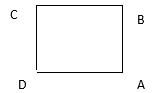(a) 0,K,$K(2+\frac{1}{\sqrt {2}})$,$2K(2+\frac{1}{\sqrt2})$
(b) 0,K,$K(2-\frac{1}{\sqrt2})$,$2K(2-\frac{1}{\sqrt2})$
(c) 0,K,2K,4K
(d) 0,K,$K(2+\frac{1}{\sqrt2})$,$4K(2+\frac{1}{\sqrt2})$
Where $K=\frac{Q^2}{4\pi\varepsilon_0}$

When A only is present
Energy is Zero
When B is placed
EE=$\frac{1}{4\pi\varepsilon_0}\frac{Q^2}{1}=K$

When C is placed
EE=$\frac{1}{4\pi\varepsilon_0}\frac{Q^2}{1}+\frac{1}{4\pi\varepsilon_0}\frac{Q^2}{1}+\frac{1}{4\pi\varepsilon_0}\frac{Q^2}{\sqrt2}=K(2+\frac{1}{\sqrt2})$

When D is placed

$EE=\frac{1}{4\pi\varepsilon_0}\frac{Q^2}{1}(2+\frac{1}{\sqrt2})+\frac{1}{4\pi\varepsilon_0}\frac{Q^2}{1}(2+\frac{1}{\sqrt2})=2K(2+\frac{1}{\sqrt2})$

Question 18
The electrostatic potential on the surface of a charged conducting sphere is 200V. Two statments are made in this regard:
S1 : At any point inside the sphere, electric intensity is zero.
S2 : At any point inside the sphere, the electrostatic potential is 200V.
Which of the following is a correct statement?
(a) S1 is true but S2 is false.
(b) Both S1 & S2 are false.
(c) S1 is true, S2 is also true and S1 is the cause of S2.
(d) S1 is true, S2 is also true but the statements are independant.

(c)

Question 19
Consider a uniform electric field in the $\hat{z}$ direction. The potential is a constant
(a) in all space.
(b) for any x for a given z.
(c) for any y for a given z.
(d) on the x-y plane for a given z.

(b),(c) and (d)

Question 20
In a region of constant potential
(a) the electric field is uniform
(b) the electric field is zero
(c) there can be no charge inside the region.
(d) the electric field shall necessarily change if a charge is placed outside the region.

(b) and (c)

## Paragraph Based Questions

(C) Two spherical cavities ( A and B) of radii x and y are hollowed out from the interior of a conducting sphere of radius R. Charge $q_1$ and $q_2$ charges are placed at center of cavities A and B. The situation is depicted in the below fig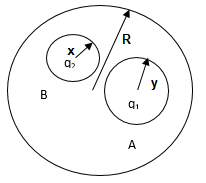Question 21
Find the surface charge densities at A, B and outer surface of the sphere
(a) $\frac{-q_1}{4\pi x^2},\frac{-q_2}{4\pi y^2},\frac{q_1+q_2}{4\pi R^2}$
(b) $\frac{q_1}{4\pi x^2},\frac{q_2}{4\pi y^2},\frac{-(q_1+q_2)}{4\pi R^2}$
(c) $\frac{-q_1}{4\pi y^2},\frac{-q_2}{4\pi x^2},\frac{q_1+q_2}{4\pi R^2}$
(d) None of these

The charge distribution will be like in the below figure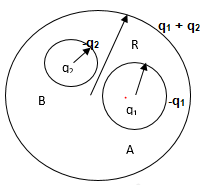It is clear from the figure
$\sigma_R=\frac{q_1+q_2}{4\pi R^2}$
$\sigma_A=\frac{-q_1}{4\pi x^2}$
$\sigma_B=\frac{-q_2}{4\pi y^2}$

Question 22
What is the electric field outside the conductor at distance r from the center of large sphere
(a) $\frac{1}{4\pi\varepsilon_0}\frac{q_1}{r^2}\mathbf{r}$
(b) $\frac{1}{4\pi\varepsilon_0}\frac{q_1+q_2}{r^2}\mathbf{r}$
(c) $\frac{1}{4\pi\varepsilon_0}\frac{q_2}{r^2}\mathbf{r}$
(d) $\frac{1}{4\pi\varepsilon_0}\frac{q_1-q_2}{r^2}\mathbf{r}$
Where r is the unit vector from center of large sphere

Electric field outside will be due to the surface charges on the outer surface only.
So

$\mathbf{E}=\frac{1}{4\pi\varepsilon_0}\frac{q_1+q_2}{r^2}\mathbf{r}$

Question 23
What is the field inside the cavity A and cavity?
(a) $\frac{1}{4\pi\varepsilon_0}\frac{q_1}{r_A^2}\mathbf{r_A},\frac{1}{4\pi\varepsilon_0}\frac{q_2}{r_B^2}\mathbf{r_B}$
(b) $\frac{1}{4\pi\varepsilon_0}\frac{q_1}{(r_A-x)^2}\mathbf{r_A},\frac{1}{4\pi\varepsilon_0}\frac{q_2}{(r_B-y)^2}\mathbf{r_B}$
(c) zero, zero
(d) None of these
Where rA and rB are the unit vector from center of cavities respectively

Draw a Gaussian spherical surface inside the cavity A of radius r
So
$E \times 4\pi r^2=\frac{q_1}{\varepsilon_0}$
Or
$E=\frac{1}{4\pi\varepsilon_0}\frac{q_1}{r^2}$
Similarly for cavity B,

Question 24
What if a third charge is placed near the conductor?
(a) Surface charge on conductor will change
(b) Electric field inside the cavity will change
(c) Electric field inside the conductor will change
(d) Surface charge on cavity will change

Option (a)
There will be no effect in cavity

Question 25
If the potential of the conductor is 1 V, find the potential at Point N at the distance r from the center of the cavity A ( r < x)
(a) $1+\frac{q_1}{4\pi\varepsilon_0}\left[\frac{1}{r}-\frac{1}{x}\right]$
(b) $1+\frac{q_1}{4\pi\varepsilon_0}\left[\frac{1}{x}-\frac{1}{r}\right]$
(c) $1-\frac{q_1}{4\pi\varepsilon_0}\left[\frac{1}{r}-\frac{1}{x}\right]$
(d) None of these

Potential at point N= Potential of the conductor + Potential due to charge q1 at center + Potential due to induced charges at inner surface
$=1+\frac{q_1}{4\pi\varepsilon_0}\frac{1}{r}+\frac{-q_1}{4\pi\varepsilon_0}\frac{1}{x}$
$=1+\frac{q_1}{4\pi\varepsilon_0}\left[\frac{1}{r}-\frac{1}{x}\right]$

(D) Three concentric spherical conductors A, B, C are arranged as shown in the figure. The radius of the shell A, B ,C are $R_1$, $R_2$ and $R_3$ respectively. Charge Q, 2Q and 3Q are given the spherical shell A, B and C respectively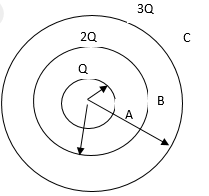Question 26
Find the Surface charge density of the outer surface of shell C
(a) $\frac{3Q}{2\pi\varepsilon_0R_3^2}$
(b) $\frac{Q}{2\pi\varepsilon_0R_3^2}$
(c) $\frac{3Q}{4\pi\varepsilon_0R_3^2}$
(d) $\frac{3Q}{\pi\varepsilon_0R_3^2}$

As charges get induced on the inner and outer surface because of the conductor A and B,
Total charge on the outer surface will be =Q+2Q+3Q=6Q
So surface charge density=
$\frac{6Q}{4\pi\varepsilon_0R_3^2}=\frac{3Q}{2\pi\varepsilon_0R_3^2}$

Option(a) is correct

Question 27
Which of these is true for potential?
(a) Potential at point $R_2 < R < R_3$ is given by
$\frac{Q}{4\pi\varepsilon_0}\left[\frac{3}{R}+\frac{3}{R_3}\right]$
(b) Potential of the outer surface of the shell C is
$\frac{Q}{4\pi\varepsilon_0}\left[\frac{6}{R_3}\right]$
(c) Potential at the center of the system is
$\frac{Q}{4\pi\varepsilon_0}\left[\frac{1}{R_1}+\frac{2}{R_2}+\frac{3}{R_3}\right]$
(d) None of these

Option (a) ,(b) and (c) are correct

Potential can be calculated as

Potential at point $R_2 < R < R_3$
= Potential due to Shell A at distance R+ Potential due to Shell B at distance R + potential due to shell C
$=\frac{1}{4\pi\varepsilon_0}\frac{Q}{R}+\frac{1}{4\pi\varepsilon_0}\frac{2Q}{R}+\frac{1}{4\pi\varepsilon_0}\frac{3Q}{R_3}$

$=\frac{Q}{4\pi\varepsilon_0}\left[\frac{3}{R}+\frac{3}{R_3}\right]$

Potential at the center of the system is
= Potential due to Shell A at center+ Potential due to Shell B at center +Potential due to shell C at center
$=\frac{Q}{4\pi\varepsilon_0}\left[\frac{1}{R_1}+\frac{2}{R_2}+\frac{3}{R_3}\right]$

Potential of the outer surface of the shell C is
= Potential due to Shell A at distance R3+ Potential due to Shell B at distance R3 + potential due to shell C
$=\frac{Q}{4\pi\varepsilon_0}\left[\frac{6}{R_3}\right]$

Question 28
Find the electric Field at a point $R > R_3$
(a) $\frac{3Q}{2\pi\varepsilon_0R^2}$
(b) $\frac{3Q}{4\pi\varepsilon_0R^2}$
(c) $\frac{3Q}{\pi\varepsilon_0R^2}$
(d) None of these

Draw a gaussian surface at distance R from center, Applying gauss law

$E=\frac{3Q}{2\pi\varepsilon_0R^2}$
So option (a) is correct.